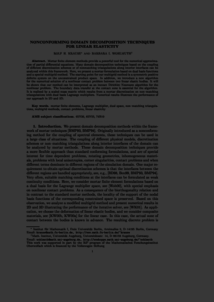Repository: Freie Universität Berlin, Math Department

# Nonconforming decomposition methods: Techniques for linear elasticity

Krause, R. and Wohlmuth, B. (2000) Nonconforming decomposition methods: Techniques for linear elasticity. East-West Journal of Numerical Mathematics, 8 (3). pp. 177-206. ISSN 1569-3953; 0928-0200Preview

929kB

Official URL: https://dx.doi.org/10.1515/jnum

## Abstract

Mortar finite element methods provide a powerful tool for the numerical approximation of partial differential equations. Many domain decomposition techniques based on the coupling of different discretization schemes or of nonmatching triangulations along interior interfaces can be analyzed within this framework. Here, we present a mortar formulation based on dual basis functions and a special multigrid method. The starting point for our multigrid method is a symmetric positive deﬁnite system on the unconstrained product space. In addition, we introduce a new algorithm for the numerical solution of a nonlinear contact problem between two linear elastic bodies It will be shown that our method can be interpreted as an inexact Dirichlet-Neumann algorithm for the nonlinear problem. The boundary data transfer at the contact zone is essential for the algorithm. It is realized by a scaled mass matrix which results from a mortar discretization on non-matching triangulations with dual basis Lagrange multipliers. Numerical results illustrate the performance of our approach in 2D and 3D.

Item Type: Article Mathematical and Computer Sciences > Mathematics > Numerical Analysis Department of Mathematics and Computer Science > Institute of Mathematics 1851 Ekaterina Engel 16 Apr 2016 19:47 03 Mar 2017 14:42

Repository Staff Only: item control page